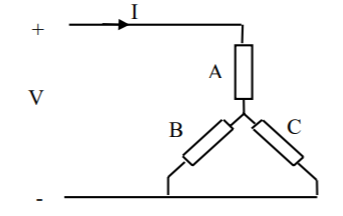# Some questions about resistance in BLDC motors

I’m currently choosing motors for a wheeled robot project im working on, and had a few questions that I wondered if anyone here could help answer.

Basically its about resistance and how it relates to the maximum continuous and peak torque.

If i wanted to use V=IR and P=I^2*R to calculate the current in the motor and the heat produced for some current, is the R that i should plug in just the phase resistance? then is that I the same one I can plug in to the torque = 8.3 * I / Kv formula?

I’m sorry im just confused because there are so many similar but different values like phase voltage vs line-to-line voltage, etc etc

thanks for any help

Yep, that all sounds right to me. Especially the part about it being confusing1 Like

Since it is a 3-phase motor, the ohmic power loss is actually `P = 3/2 * I^2 * R` if R is the phase resistance. That is because the other phases also have current in them. Best way to visualize it is with the current angle aligned to a phase like this:Note that the ODrive will limit the current, so if you try to use `V=IR` to calculate the current and you use 24V or 48V or similar for V, you will get much higher currents than what will actually occur with the active current limiting.